Friday, October 22, 2021
Home > IMO > Pre RMO Previous Year 2015 Question Paper With Solutions

# Pre RMO Previous Year 2015 Question Paper With Solutions# Pre RMO Previous Year 2015 Question Paper With Solutions

In this post, you will get Pre RMO Previous Year 2015 Question Paper With Solutions.

Set APre RMO Previous Year 2015 Question Paper Question No 1:

A man walks a certain distance and rides back inhours; he could ride both ways inhours. How many hours would it take him to walk both ways?

Pre RMO Previous Year 2015 Question Paper Solution of Question No 1: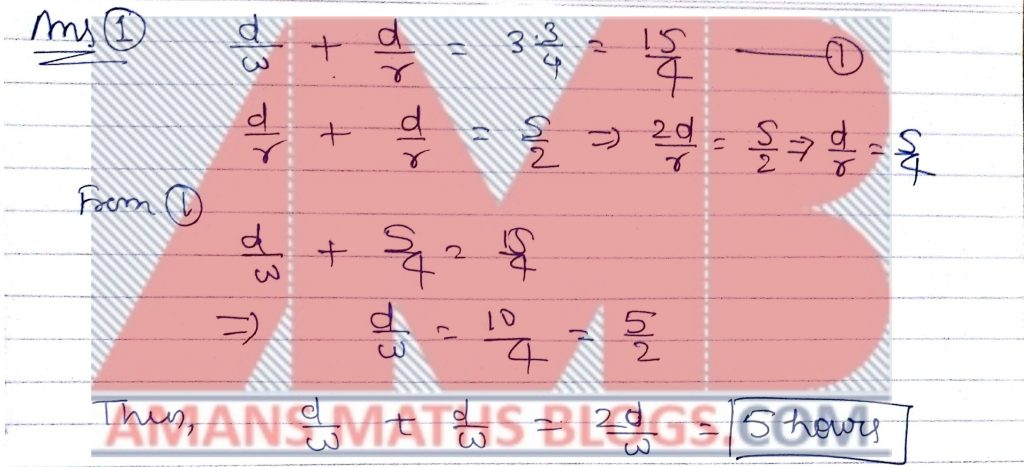Pre RMO Previous Year 2015 Question Paper Question No 2:

Positive integers a and b are such that a + b = a/b + b/a. What is the value of a2 + b2?

Pre RMO Previous Year 2015 Question Paper Solution of Question No 2: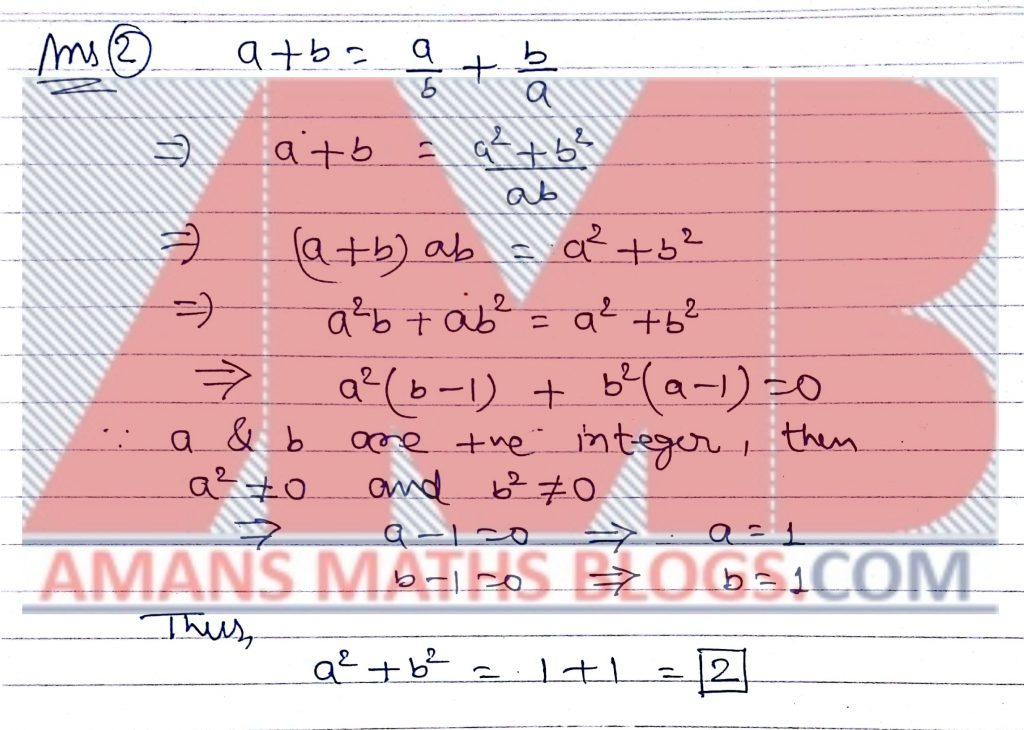Pre RMO Previous Year 2015 Question Paper Question No 3:

The equations x2 − 4x + k = 0 and x2 + kx − 4 = 0, where k is a real number, have exactly one common root. What is the value of k?

Pre RMO Previous Year 2015 Question Paper Solution of Question No 3: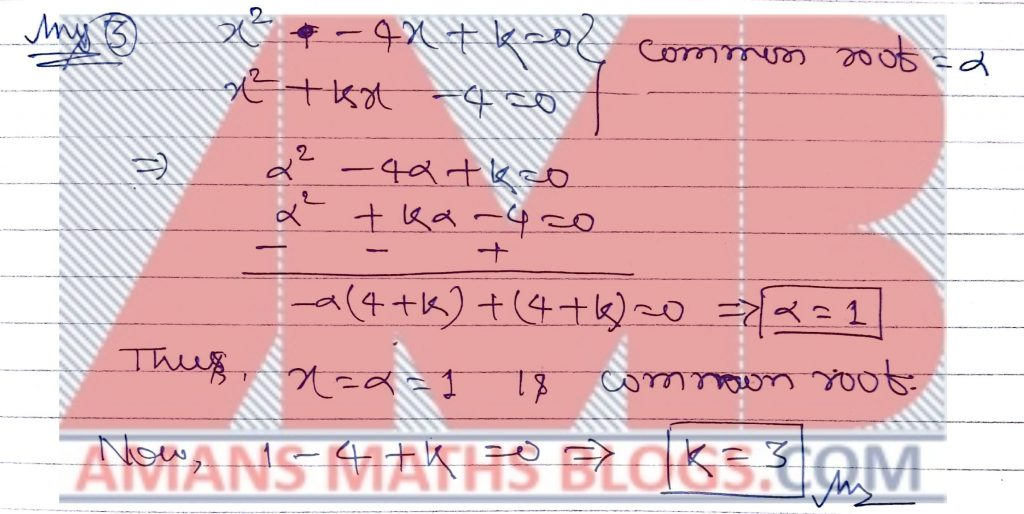Pre RMO Previous Year 2015 Question Paper Question No 4:

Let P(x) be a non-zero polynomial with integer coefficients. If P(n) is divisible by n for each positive integer n, what is the value of P(0)?

Pre RMO Previous Year 2015 Question Paper Solution of Question No 4: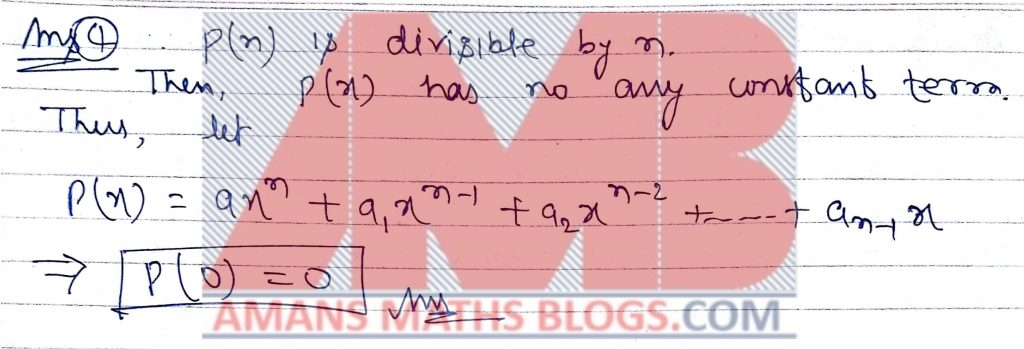Pre RMO Previous Year 2015 Question Paper Question No 5:

How many line segments have both their endpoints located at the vertices of a given cube?

Pre RMO Previous Year 2015 Question Paper Solution of Question No 5: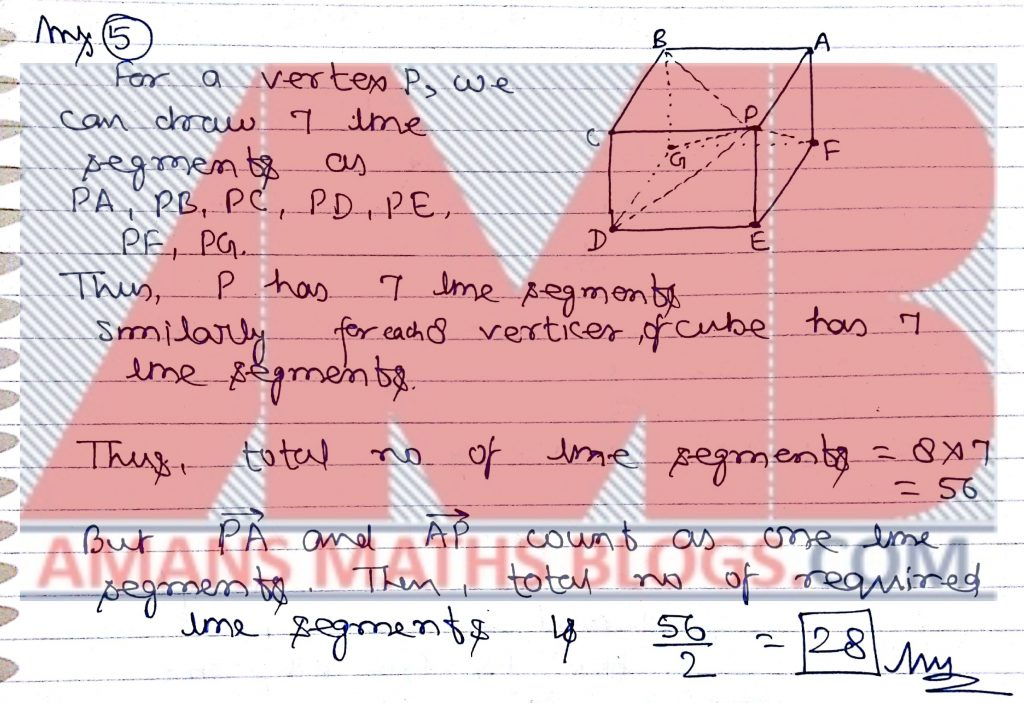Pre RMO Previous Year 2015 Question Paper Question No 6:

Let E(n) denote the sum of the even digits of n. For example, E(1243) = 2 + 4 = 6. What is the value of E(1) + E(2) + E(3) + · · · + E(100)?

Pre RMO Previous Year 2015 Question Paper Solution of Question No 6: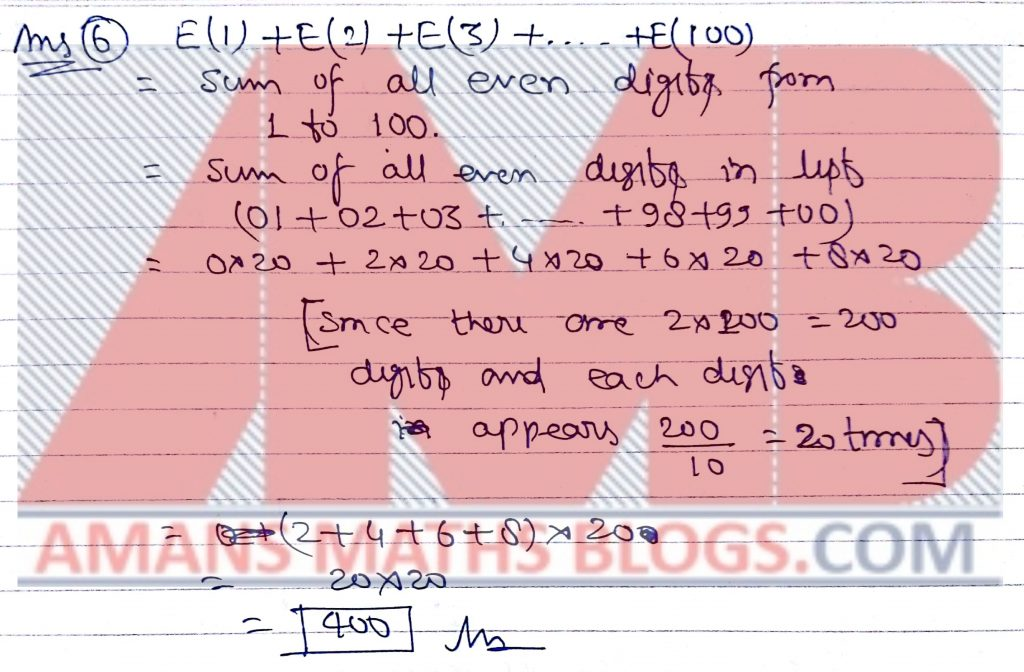Pre RMO Previous Year 2015 Question Paper Question No 7:

How many two-digit positive integers N have the property that the sum of N and the number obtained by reversing the order of the digits of N is a perfect square?

Pre RMO Previous Year 2015 Question Paper Solution of Question No 7: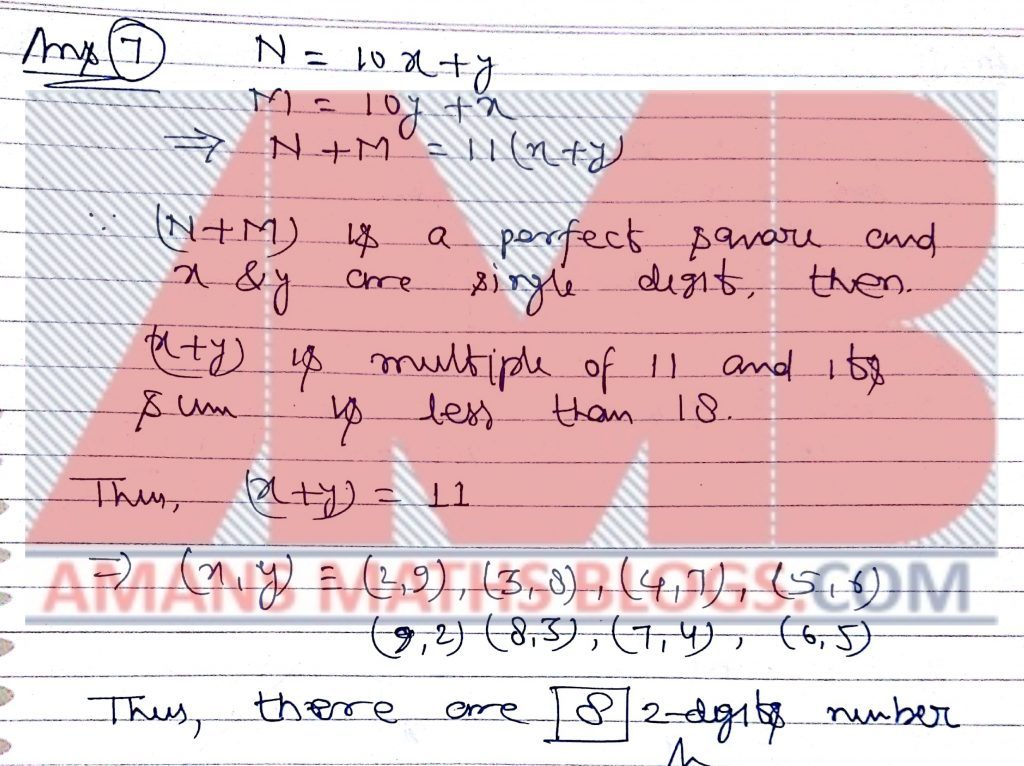Pre RMO Previous Year 2015 Question Paper Question No 8:

The figure below shows a broken piece of a circular plate made of glass.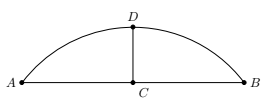C is the midpoint of AB, and D is the midpoint of arc AB. Given that AB = 24 cm and CD = 6 cm, What is the radius of the plate in centimeters? (The figure is not drawn to scale.)

Pre RMO Previous Year 2015 Question Paper Solution of Question No 8: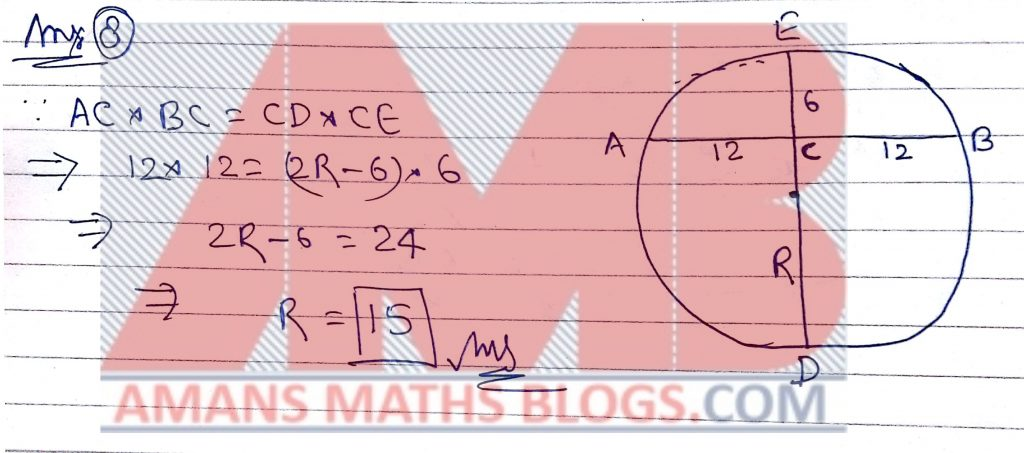Pre RMO Previous Year 2015 Question Paper Question No 9:

A 2 × 3 rectangle and a 3 × 4 rectangle are contained within a square without overlapping at any interior point, and the sides of the square are parallel to the sides of the two given rectangles. What is the smallest possible area of the square?

Pre RMO Previous Year 2015 Question Paper Solution of Question No 9: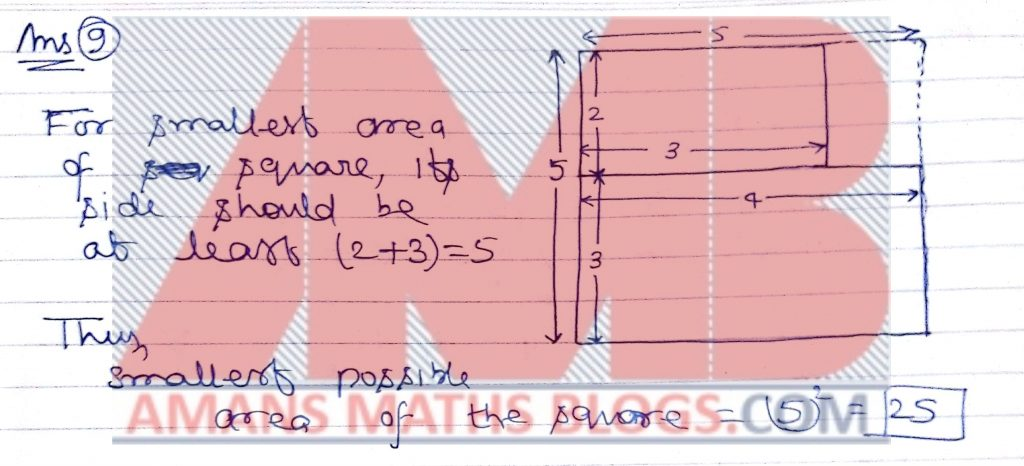Pre RMO Previous Year 2015 Question Paper Question No 10:

What is the greatest possible perimeter of a right-angled triangle with integer side lengths if one of the sides has length 12?

Pre RMO Previous Year 2015 Question Paper Solution of Question No 10: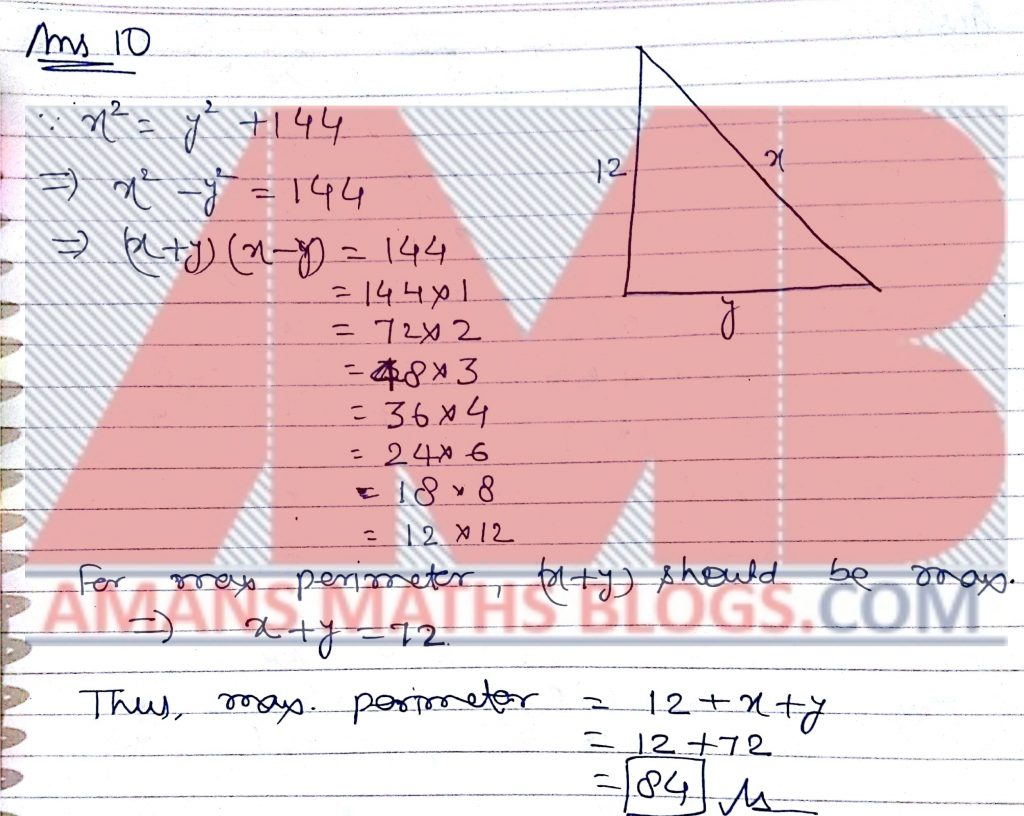Pre RMO Previous Year 2015 Question Paper Question No 11:

In rectangle ABCD, AB = 8 and BC = 20. Let P be a point on AD such that 6 BP C = 90◦. If r1, r2, r3 are the radii of the incircles of triangles AP B, BP C and CP D, what is the value of r1 + r2 + r3?

Pre RMO Previous Year 2015 Question Paper Solution of Question No 11: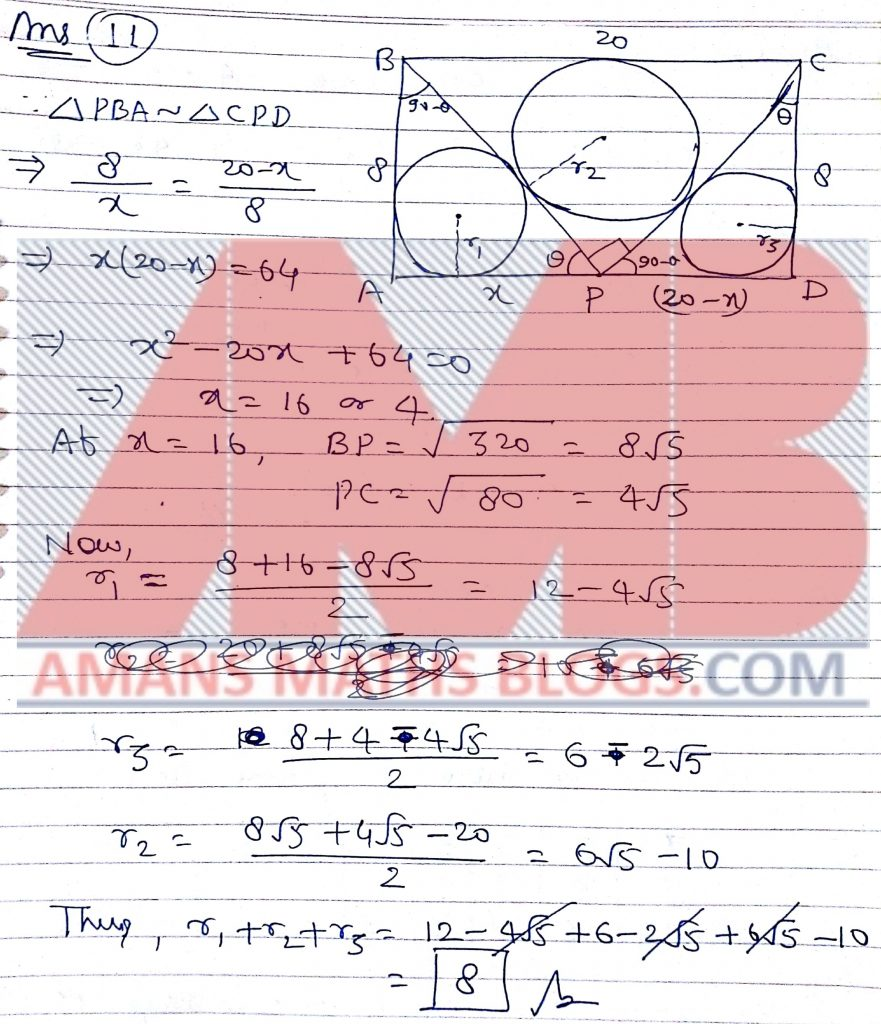Pre RMO Previous Year 2015 Question Paper Question No 12:

Let a, b, and c be real numbers such that a − 7b + 8c = 4 and 8a + 4b − c = 7. What is the value of a2 − b2 + c2?

Pre RMO Previous Year 2015 Question Paper Solution of Question No 12: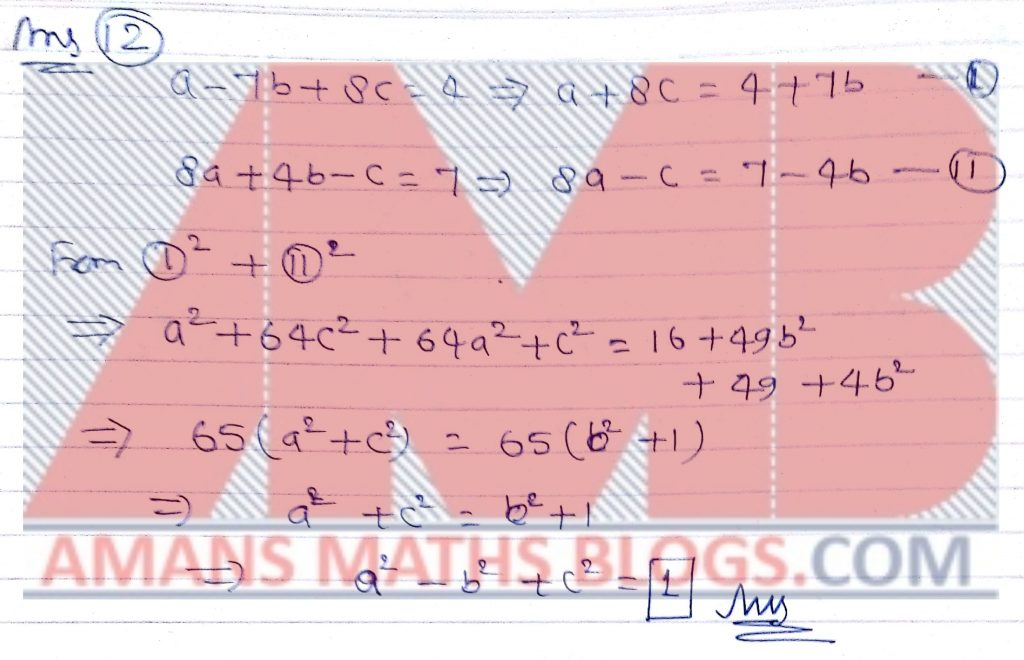Pre RMO Previous Year 2015 Question Paper Question No 13:

Let n be the largest integer that is the product of exactly 3 distinct prime numbers, x, y and 10x + y, where x and y are digits. What is the sum of the digits of n?

Pre RMO Previous Year 2015 Question Paper Solution of Question No 13: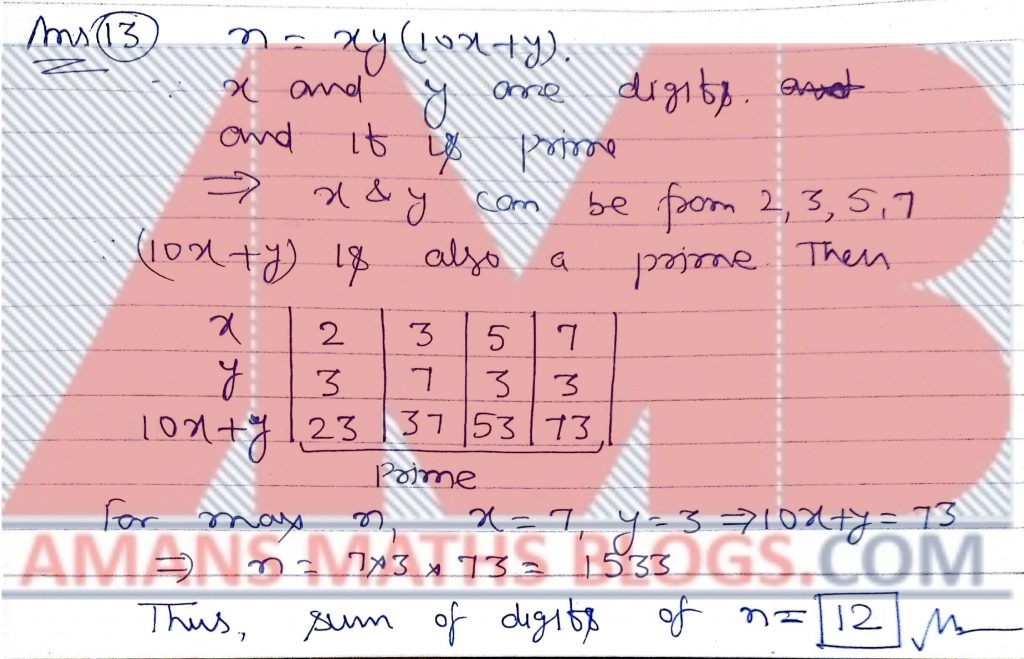Pre RMO Previous Year 2015 Question Paper Question No 14:

At a party, each man danced with exactly four women and each woman danced with exactly three men. Nine men attended the party. How many women attended the party?

Pre RMO Previous Year 2015 Question Paper Solution of Question No 14: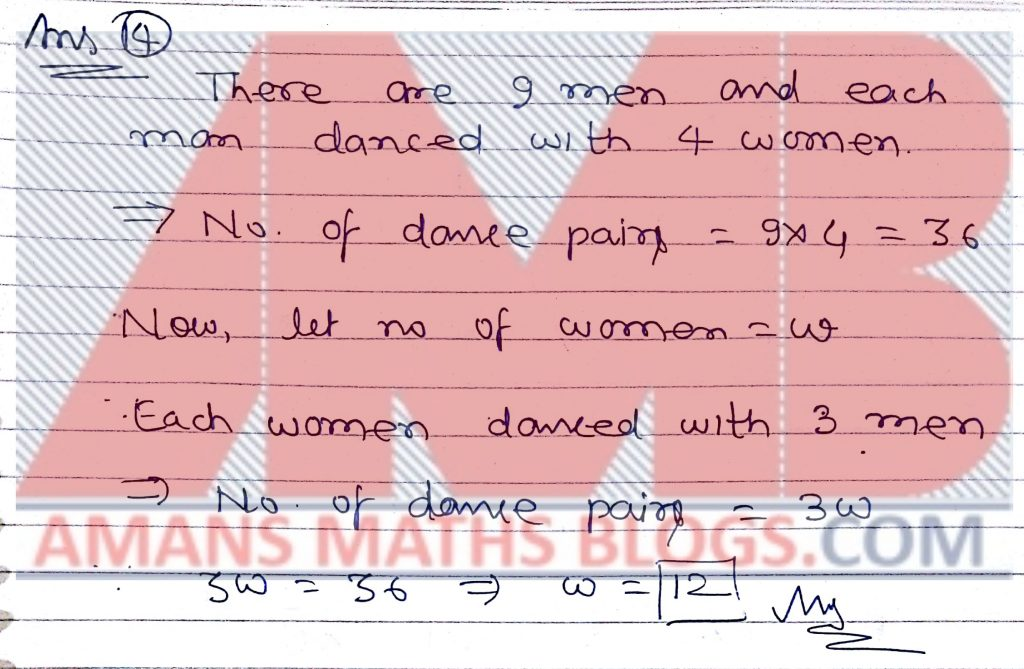Pre RMO Previous Year 2015 Question Paper Question No 15:

If 3x + 2y = 985 and 3x − 2y = 473, what is the value of xy?

Pre RMO Previous Year 2015 Question Paper Solution of Question No 15: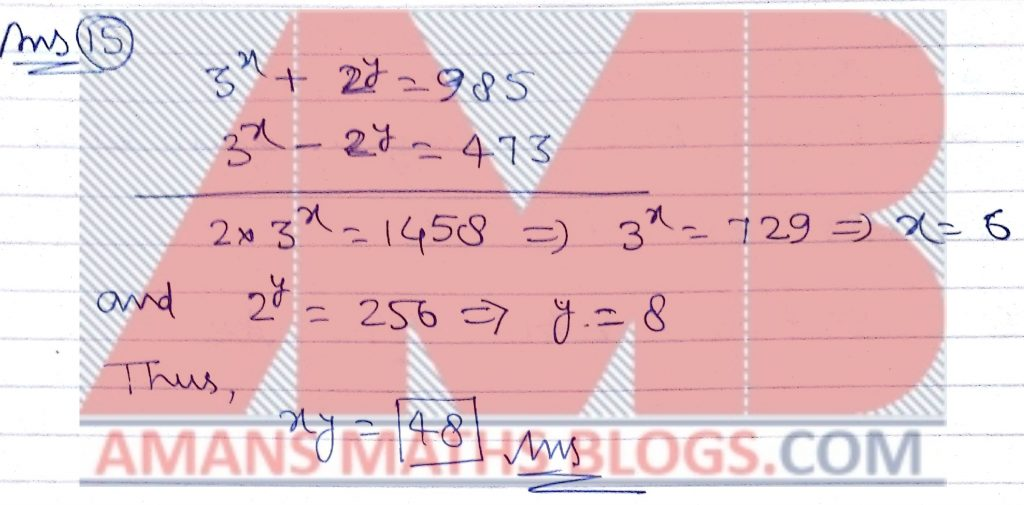Pre RMO Previous Year 2015 Question Paper Question No 16:

In acute-angled triangle ABC, let D be the foot of the altitude from A, and E be the midpoint of BC. Let F be the midpoint of AC. Suppose angle BAE = 40◦. If angle DAE = angle DF E, what is the magnitude of angle ADF in degrees?

Pre RMO Previous Year 2015 Question Paper Solution of Question No 16: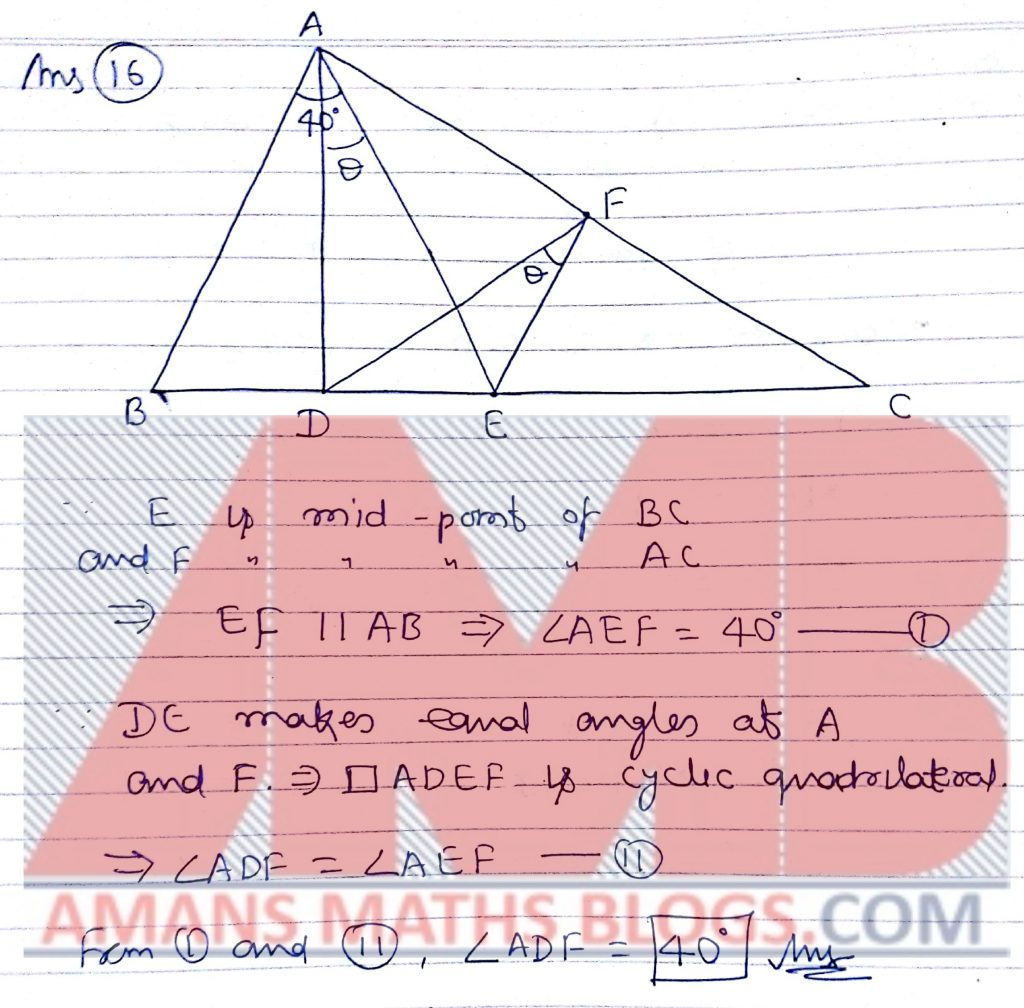Pre RMO Previous Year 2015 Question Paper Question No 17:

A subset B of the set of first 100 positive integers has the property that no two elements of B sum to 125. What is the maximum possible number of elements in B?

Pre RMO Previous Year 2015 Question Paper Solution of Question No 17: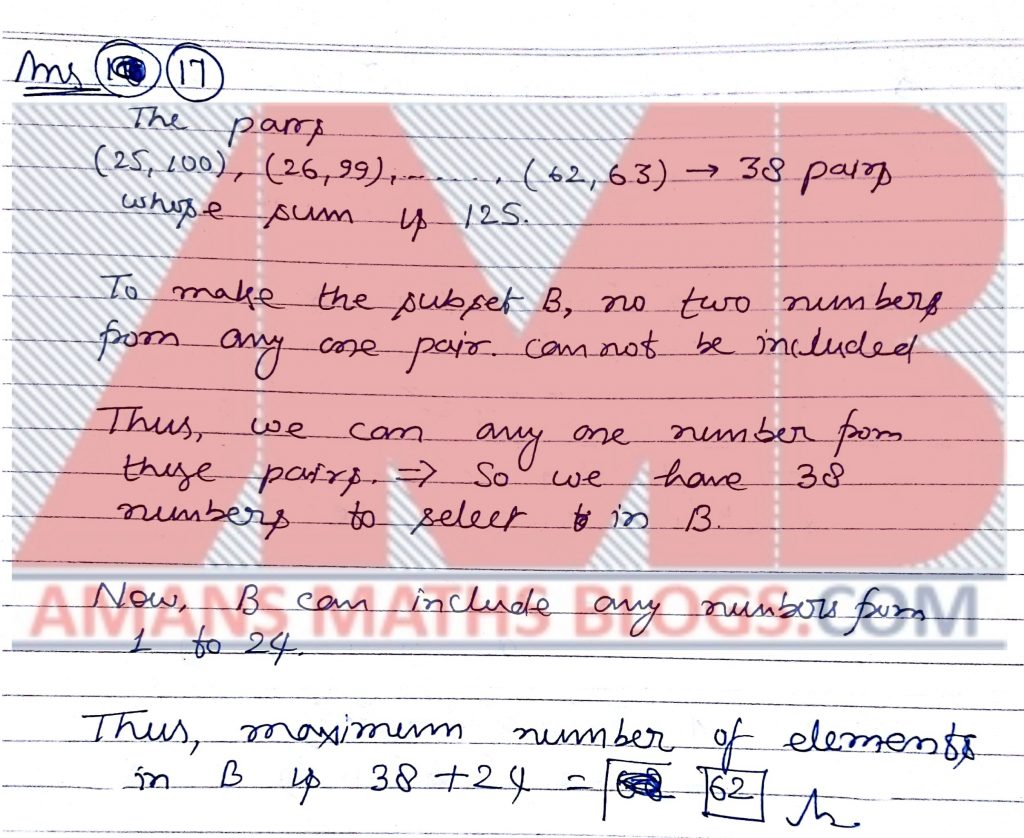Pre RMO Previous Year 2015 Question Paper Question No 18:

Let a, b and c be such that a + b + c = 0 and is defined.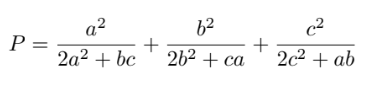What is the value of P?

Pre RMO Previous Year 2015 Question Paper Solution of Question No 18: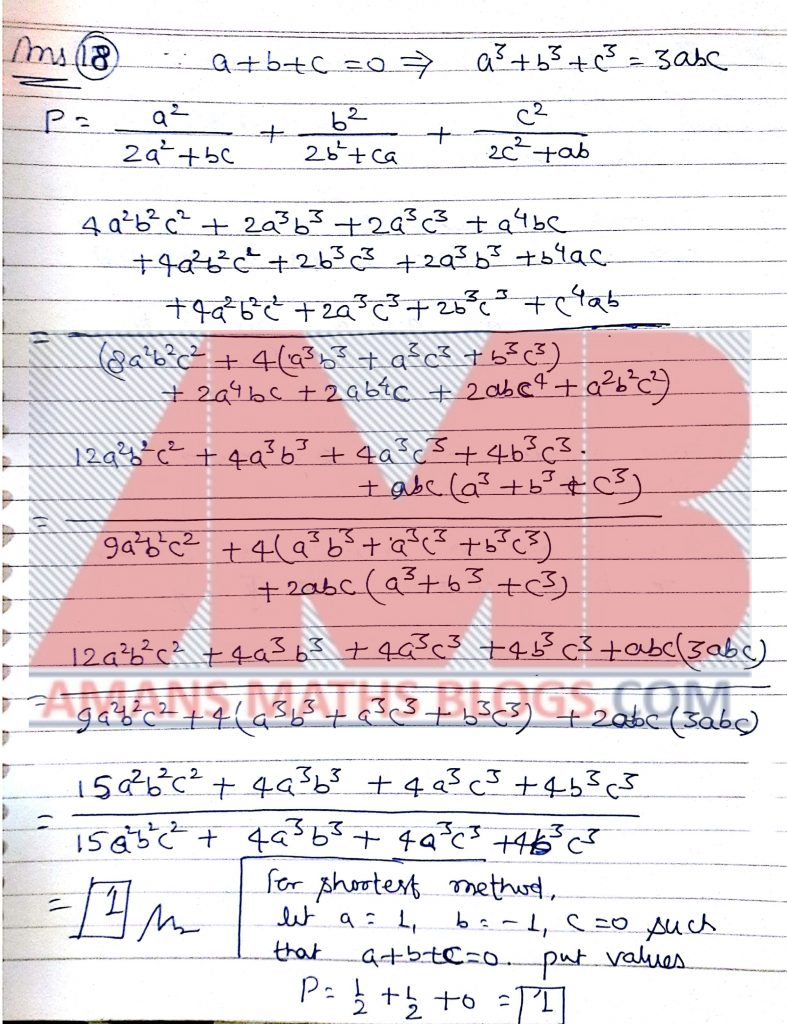Pre RMO Previous Year 2015 Question Paper Question No 20:

The digits of a positive integer n are four consecutive integers in decreasing order when read from left to right. What is the sum of the possible remainders when n is divided by 37?

Pre RMO Previous Year 2015 Question Paper with Solutions of Question No 20: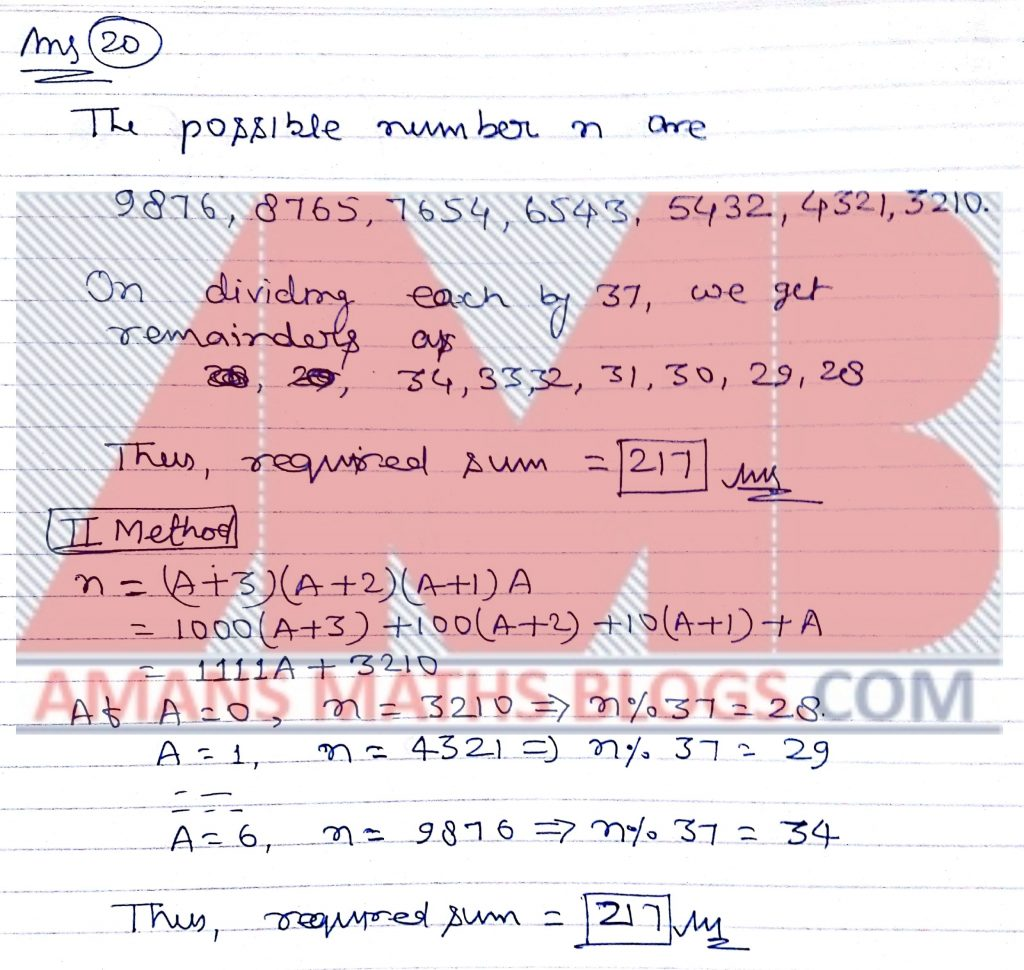Pre RMO Previous Year 2015 Question Paper With SolutionGet Combined Pre RMO 2012 to 2018 Questions Paper with Solution (Click on Image)

Set B

AMBiPi
error: Content is protected !!